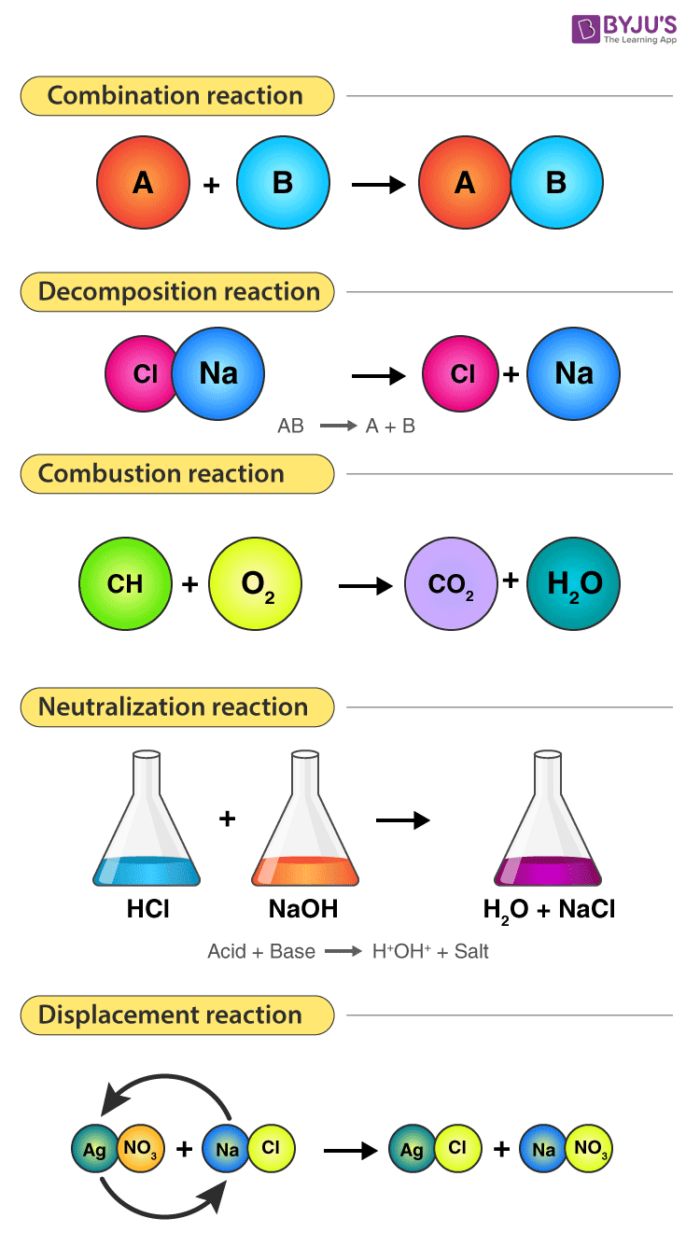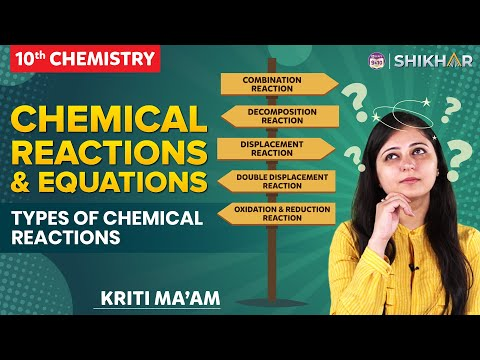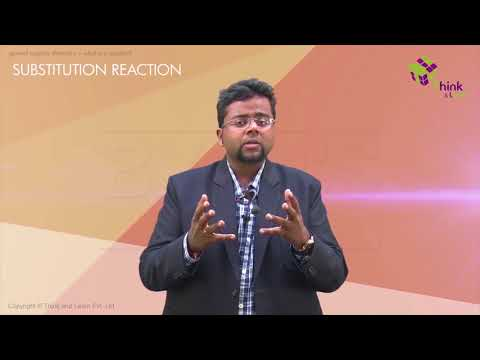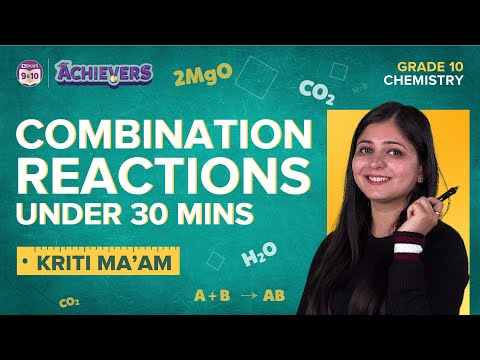# Types of Chemical Reactions

During a chemical reaction, the substances that react are known as reactants whereas the substances that are formed during a chemical reaction are known as products. Six common types of chemical reactions are discussed below.Different Types of Chemical Reactions – Combination, Decomposition, Combustion, Neutralization & Displacement Reactions

 Types of Chemical Reactions Explanation General Reaction Combination reaction Two or more compounds combine to form one compound. A + B → AB Decomposition reaction The opposite of a combination reaction – a complex molecule breaks down to make simpler ones. AB → A + B Precipitation reaction Two solutions of soluble salts are mixed resulting in an insoluble solid (precipitate) forming. A + Soluble salt B  → Precipitate + soluble salt C Neutralization reaction An acid and a base react with each other. Generally, the product of this reaction is salt and water. Acid + Base → Salt + Water Combustion reaction Oxygen combines with a compound to form carbon dioxide and water. These reactions are exothermic, meaning they give off heat. A + O2 → H2O + CO2 Displacement reaction One element takes place with another element in the compound. A + BC → AC + B

Also, check ⇒ Chemistry Concept Questions and Answers

## Chemical Reactions and Equations – Types of Chemical Reactions#### Types Of Organic Reactions#### Types of Chemical Reactions## Different Types of Chemical Reactions

The 5 primary types of chemical reactions are:

### 1. Combination Reaction

• A reaction in which two or more reactants combine to form a single product is known as a combination reaction.
• It takes the form of X + Y → XY
• Combination reaction is also known as a synthesis reaction.
• Example of combination reaction: 2Na + Cl2 → 2NaCl

## Combination Reactions### 2. Decomposition Reaction

• A reaction in which a single compound breaks into two or more simpler compounds is known as a decomposition reaction.
• It takes the form of XY → X + Y
• A decomposition reaction is just the opposite of a combination reaction.
• Example of a decomposition reaction: CaCO3 → CaO + CO2
• The reaction in which a compound decomposes due to heating is known as a thermal decomposition reaction.

### 3. Displacement Reaction

• A chemical reaction in which a more reactive element displaces a less reactive element from its aqueous salt solution.
• It takes the form X + YZ → XZ + Y
• It is also called a substitution reaction
• Example of displacement reaction: Zn + CuSO4 → ZnSO4 + Cu

### 4. Double Displacement Reaction

• A chemical reaction in which ions get exchanged between two reactants which form a new compound is called a double displacement reaction.
• It takes the form of XY + ZA → XZ + YA
• It is also called a metathesis reaction
• Example of double displacement reaction:

$$\begin{array}{l}~~~~~~~~~~~\end{array}$$
BaCl2 + Na2SO4 → BaSO4 + 2NaCl

### 5. Precipitation Reaction

• A chemical reaction that involves the formation of an insoluble product (precipitate; solid) is called Precipitation reaction.
• The reactants are soluble, but the product formed would be insoluble and separates out as a solid.
• The chemical equation by which a chemical change is described is adequate for reaction in solutions, but for reactions of ionic compounds in aqueous solution (water), the typical molecular equation has different representations.
• A molecular equation may indicate formulas of reactants and products that are not present and eliminate completely the formulas of the ions that are the real reactants and products.
• If the substance in the molecular equation that is actually present as dissociated ions are written in the form of their ions, the result is an ionic equation.

A precipitation reaction occurs when a solution, originally containing dissolved species, produces a solid, which generally is denser and falls to the bottom of the reaction vessel.

The most common precipitation reactions occurring in aqueous solution involve the formation of an insoluble ionic compound when two solutions containing soluble compounds are mixed. Consider what happens when an aqueous solution of NaCl is added to an aqueous solution of AgNO3. The first solution contains hydrated Na+ and Cl ions and the second solution, Ag+, and NO3 ions.

NaCl(s) → Na+(aq) + Cl(aq)

AgNO3(s) → Ag+(aq) + NO3(aq)

When mixed, a double displacement reaction takes place, forming the soluble compound NaNO3 and the insoluble compound AgCl. In the reaction vessel, the Ag+ and Cl ions combine, and a white solid precipitated from the solution. As the solid precipitates, the Na+ and NO3 ions remain in solution.

The overall double displacement reaction is represented by the following balanced equation:

NaCl(aq) + AgNO3(aq) → AgCl(s) + NaNO3(aq)

## Example Problem

1. When aqueous solutions of Pb(NO3)2 and KI are mixed, does a precipitate form?
2. Write a balanced equation for the precipitation reaction that occurs when aqueous solutions of copper(II) iodide and potassium hydroxide are combined.

Solution:

You are asked to predict whether a precipitate will form during a chemical reaction and to write a balanced equation for a precipitation reaction.

You are given the identity of two reactants.

1. Yes, a solid precipitate, PbI2, forms when these solutions are mixed:

Pb(NO3)2(aq) + KI(aq)  PbI2(s) + 2KNO3(aq)

2. The two products of the reaction are insoluble copper (II) hydroxide and soluble potassium iodide.

CuI2(aq) + 2 KOH(aq)  Cu(OH)2(s) + 2 KI(aq)

## Frequently Asked Questions – FAQs

Q1

### How do you identify a chemical reaction?

A chemical reaction is typically followed by physical signs that are readily detected, such as heat and light emission, precipitate formation, gas evolution, or a change of appearance.

Q2

### How do you identify physical and chemical changes?

The shape or form of the matter varies through a physical transition, but the sort of matter in the material does not. In a chemical shift, however, the type of matter shifts and at least one new material with new properties is created. There is no straight cut of the gap between physical and chemical transition.

Q3

### Why do we write a chemical equation?

The purpose of writing a balanced chemical equation is to explain the occurring reactants (starting material) and products (end results). The ratios in which they answer so that you can measure the amount of reactants you need and the amount of goods you can make.

Q4

### What is chemical reaction and equation?

A chemical equation is the symbolic representation in the form of symbols and formulas of a chemical reaction in which the reactant entities on the left-hand side and the product entities on the right-hand side are given.

Q5

### What is the skeleton equation?

A skeleton equation is when each product that takes part in the reaction is written with the chemical formulas describing the chemical reaction. Examples: The term equation: oxygen + methane. Dioxide with carbon + Vapour.

Test Your Knowledge On Types Of Chemical Reactions!

1. Very nice and helpful 😊😊🌟🌟🦄🌟🌟

• VERY HELPFUL AND GREAT EFFORTS TAKEN

2. Thanks for this 🤗🤗🤗

3. Thank you so much, this is very helpful especially in my course.

4. thank you this is so helpul

5. Thanks for this

6. This was very helpful for me. Thank you.

7. Thank you so much very helpful🥰🥰